Updated date:

# Using Excel COUNTIF Function in Frequency Distribution

Author:

## Using Excel to Find Relative Distribution

In the study of business statistics, one of the first things you will learn is the difference between qualitative and quantitative data. The difference between the two is one of labels and numbers. Qualitative data is data that consist of names or categories of like items. Quantitative data on the other hand is data consisting of the number of items within a list of data, etc.

Secondly, the next thing you may learn is something called frequency distribution. Frequency distribution is how many times an item shows up within a list of data. The item itself would be considered qualitative data however its frequency within a list would be the number of times that item shows up which are considered quantitative.

With the above said, I am going to show you how you can take a list of qualitative data imported into Excel and find out how many times each item shows up within that list by using Excel’s COUNTIF function. For this project, I am using EXCEL 2010; however, this should work for any later versions of EXCEL.

## First some more definitions

Before I show you how to use the COUNTIF function, I am going to expand on two new definitions that are derived from the subject of frequency distribution.

First there is what the subject of business statistics calls relative frequency distribution. Relative means that for each item or category within a list of data, there is the number of times that that item shows up in the list.

Secondly, there is something known as percent frequency distribution which is about the same as relative frequency but given in a percent. Basically, the percent distribution is the relative number of items multiplied by 100.

To show how to use the above definitions in Excel, I am going to pretend that I am working at a Volkswagen auto dealership and I am given a list of different models that where sold over the last few months. The goal is to create a frequency distribution analysis from Excel that will allow me to find out what cars sell the most. In turn, I will be able to use this information for ordering more cars to sell from the manufacture in the near future.

## How to use Excel’s COUNTIF function

First of all, I know from working with this company, we only sell five different models of cars. In this case, we sell Volkswagen’s Golf Mk6, Jetta, EOS, Passat B6, and Passat B7. We also have our list of all the cars sold within the last few months imported into Excel. So, all we need to do now is to create our five categories, one for each model, and use the COUNTIF function to get a frequency of times each model shows up in the list. With that said, here is our Excel worksheets entering the COUNTIF function for our first model (EOS):

## Figure 1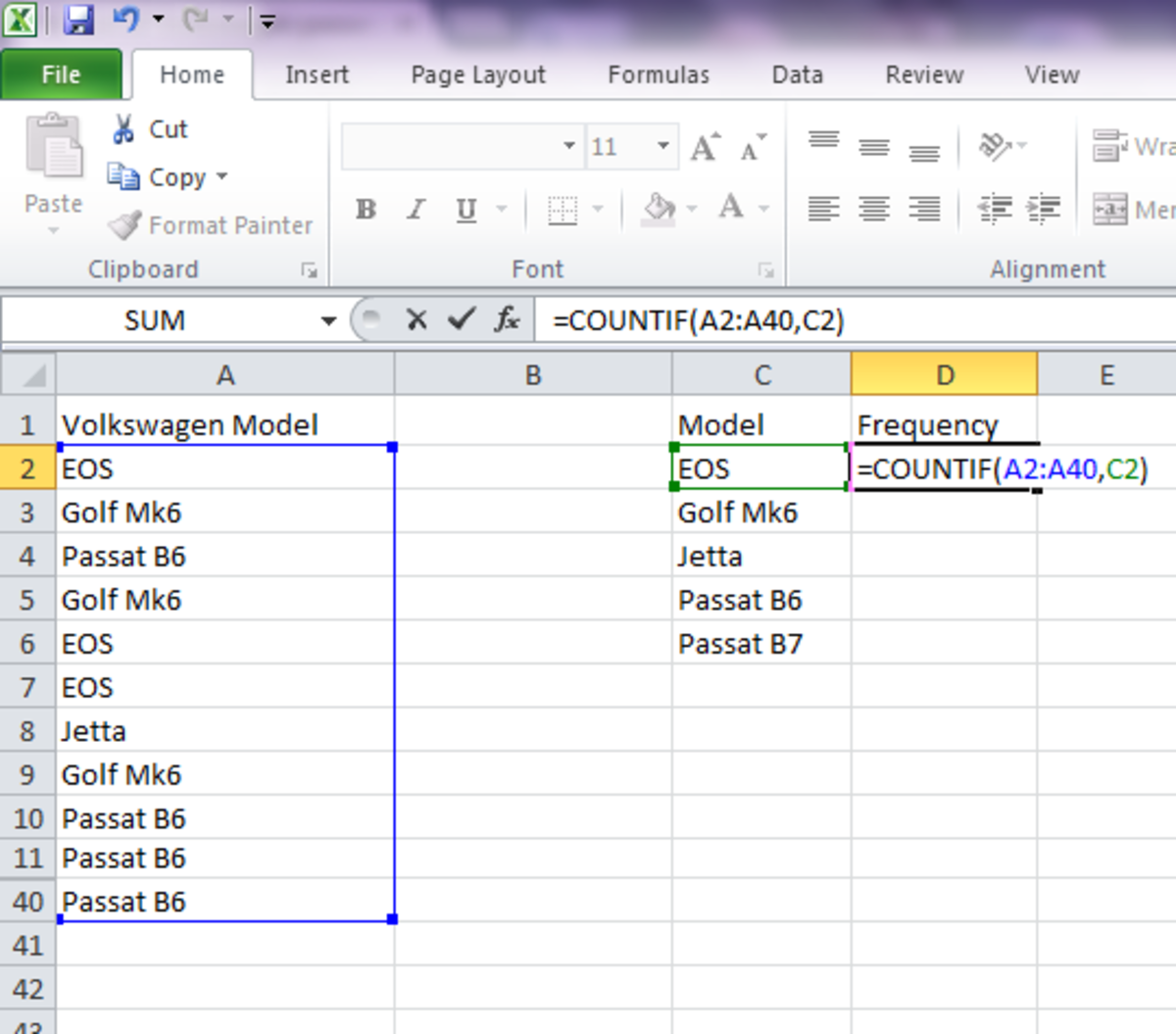To get the relative distribution of each item in a list, use Excel's COUNTIF() function.

As you can see from the graphic above, we are going to use the COUNTIF function to find out how many times we sold an EOS model. Within the COUNTIF function you will noticed two arguments. The first argument is the actual lists of items and the second argument is telling the function to count all items with the same name of what is typed into cell C2. By doing this, the function will go through the whole list and only count how many times it sees the word EOS.

With that said, we will also need to insert four more functions for the other models. Please note that if you copy and paste the above formula; you may come out with the wrong results. To alleviate this problem you could also type the first formula like the following:

COUNTIF(\$A\$2:\$A\$40, C2)

By typing the formula like the above, you can copy and paste the formula for the remaining categories without causing a logical error in you calculations.

After you have typed in the COUNTIF formula for each model, you will also need to get the total amount of cars that are on your list. To get this total, I decided to use the Sum function. Here is our Excel worksheet so far.

## Figure 2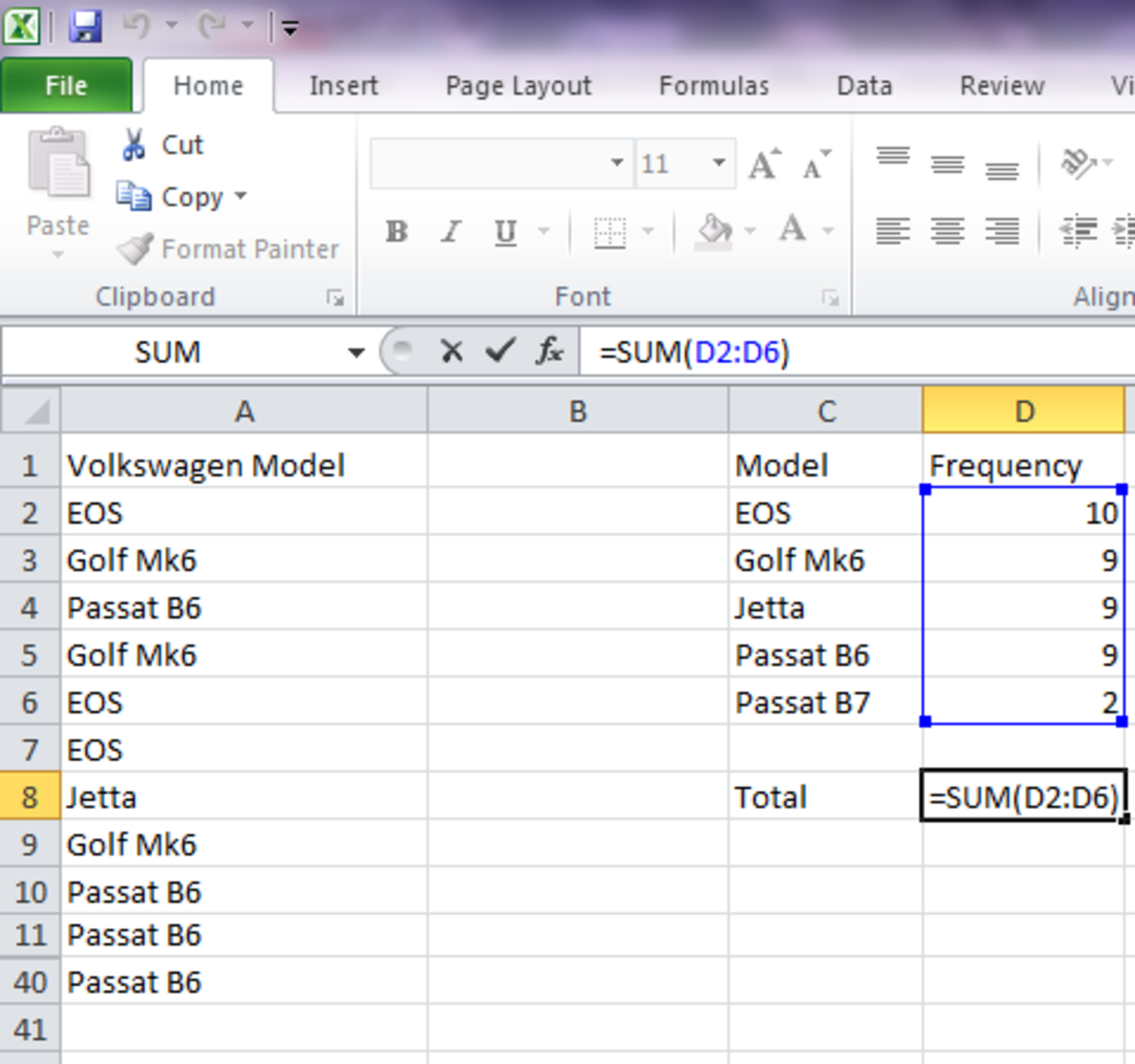Once you get the total count of each model, then use the SUM() function for the total items in the list.

Now that we have the frequency for each item in the list, we need to find its relative frequency distribution and later the percent frequency distribution. To get each item relative distribution, all we have to do is to enter for each item the formula Item/Total. In the case of the EOS model that formula would be D2/\$D\$8:

## Figure 3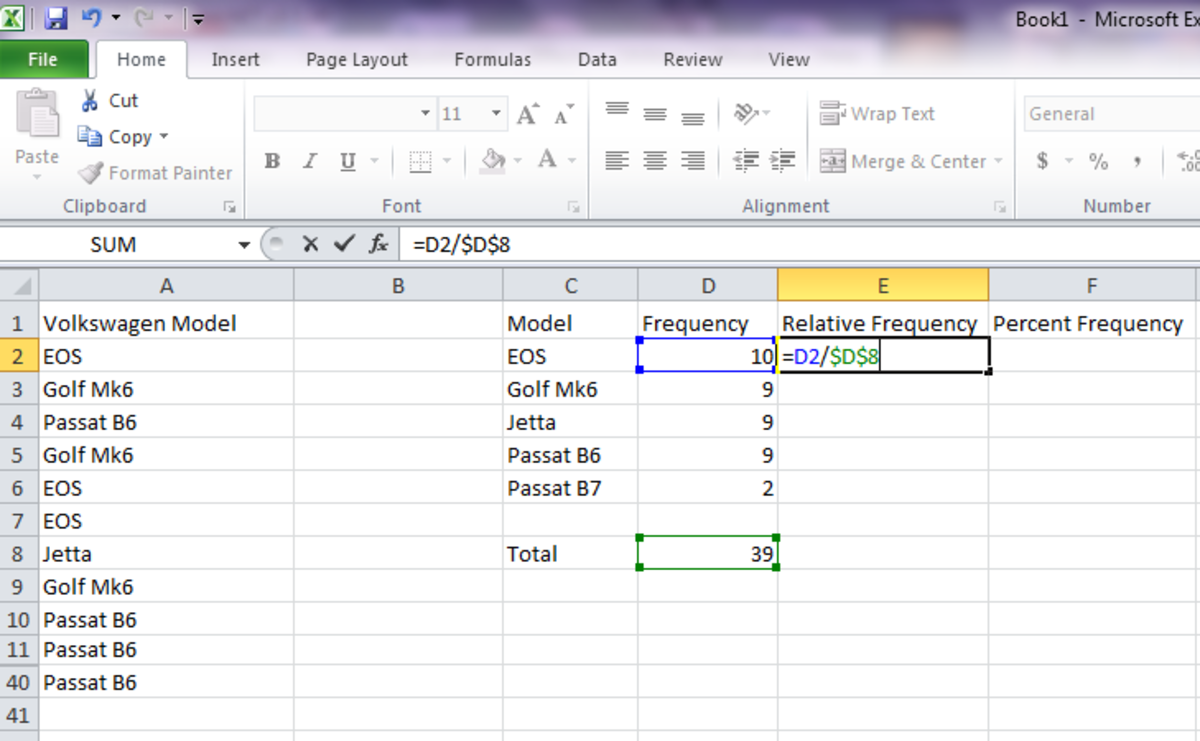Divide the relative frequency number by the total items in the list to get the relative frequency distribution.

## Figure 4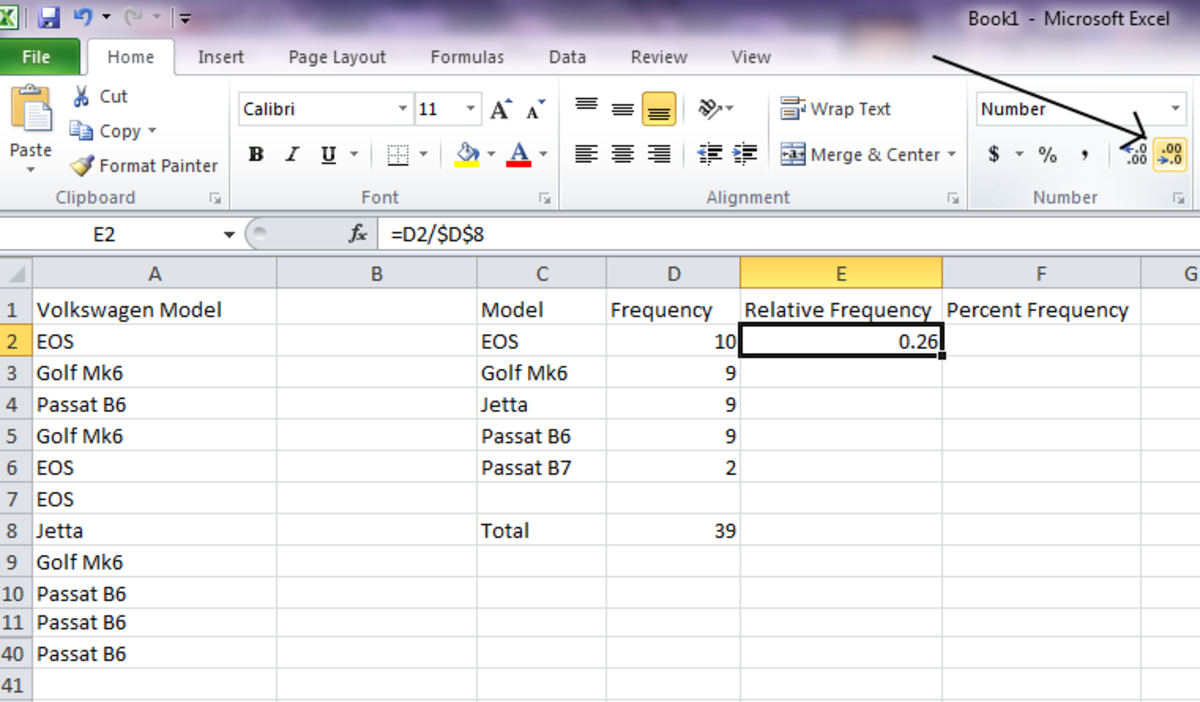Press the button in the number tab to get the number down to two decimal places.

Again, I used the \$ for the cell D8 so I can copy and paste the formula for the other models. If you do not do this, Excel will increment the number each time you paste the formula to a different cell.

Also, after you use this formula, you may have notice that you will get a decimal number that goes out to eight or nine places. The desire is to only to go out a total of two decimal places. So, to fix this, I just adjust the number in Excel’s main tool bar within the Number tab.

I also used the SUM function again to get a total for the column. The reason of doing this is to see if the total comes out to 1. If it does not, then there is an error somewhere within your data.

So, after you copy and pasted the formula to the other models, you will now need to enter the formula for the percentage frequency. To get that, all you need to do is to multiply the results from the relative frequency column by 100 also I used the SUM function one more time to make sure that the total percent is indeed 100%:

## Figure 5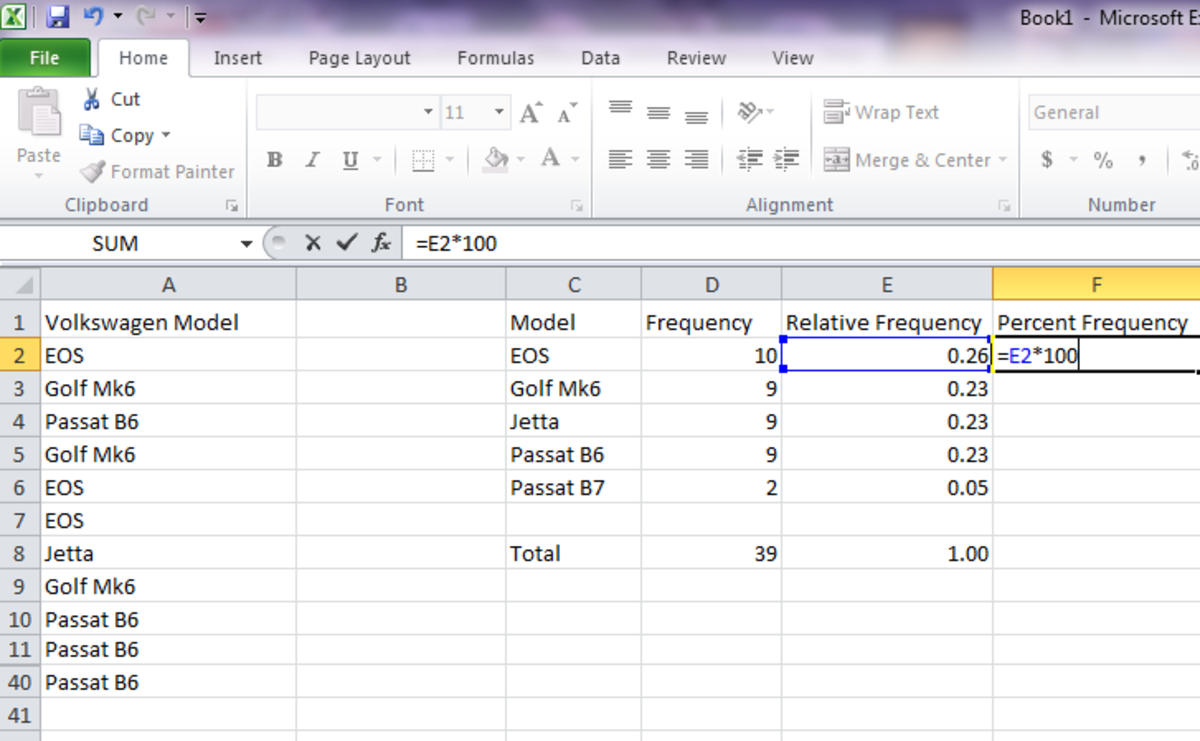To get the percent frequency, just multiply the relative frequency by 100.

When it is all done, the following worksheet would be the results:

## Figure 6

As you can see, by looking at the worksheet, the EOS is the most selling car while the Golf Mk6, Jetta, and Passat B6 are a close second. However, the Passat B7 does not seem to sell that much. Either way, this analysis gives you a good idea on how many of each model you should purchase from the manufacturer for future sells.

On a final note, I would like to do one more thing to our analysis and that is to create a bar chart. To do so, all you have to do is to highlight the Model and Frequency categories and click the insert tab to create the bar chart:

## Figure 7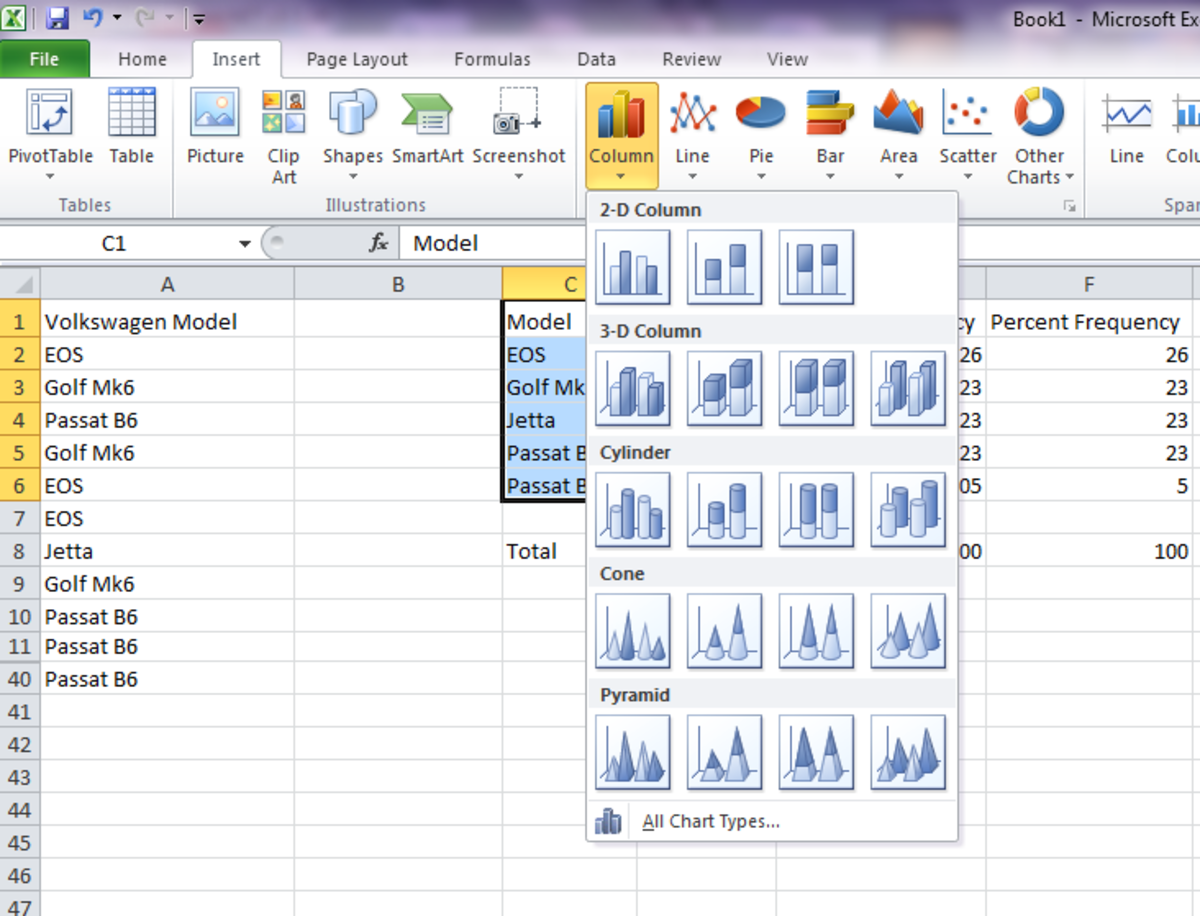To create a bar chart from your data, highlight the models and relative frequency column and then click insert tab then chart.

Then you will have the final results:

## Conclusion

That is it. Now you know how to take a list of data in Excel and to get a count of each item in the list using the COUNTIF function. We also highlighted the use of the SUM function to get the total count which in turn gave us the relative and percent distribution of each item in the list.

This works well if you only have to track a few items and you want to know what items are selling well and which are not. Excel is an excellent tool for statistical analysis and has a lot of functions to help you in making more sound decisions.